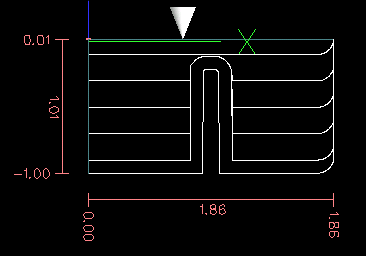## 1. What is a depth map?

A depth map is a greyscale image where the brightness of each pixel corresponds to the depth (or height) of the object at each point.

## 2. Integrating image-to-gcode with the AXIS user interface

Add the following lines to the [FILTER] section of your .ini file to make AXIS automatically invoke image-to-gcode when you open a .png, .gif, or .jpg image

```PROGRAM_EXTENSION = .png,.gif,.jpg Grayscale Depth Image
png = image-to-gcode
gif = image-to-gcode
jpg = image-to-gcode```

The standard sim/axis.ini configuration file is already configured this way.

## 3. Using image-to-gcode

Start image-to-gcode either by opening an image file in AXIS, or by invoking image-to-gcode from the terminal, as follows:

`image-to-gcode torus.png > torus.ngc`

Verify all the settings in the right-hand column, then press OK to create the gcode. Depending on the image size and options chosen, this may take from a few seconds to a few minutes. If you are loading the image in AXIS, the gcode will automatically be loaded and previewed once image-to-gcode completes. In AXIS, hitting reload will show the image-to-gcode option screen again, allowing you to tweak them.

## 4. Option Reference

### 4.1. Units

Specifies whether to use G20 (inches) or G21 (mm) in the generated g-code and as the units for each option labeled (units).

### 4.2. Invert Image

If “no”, the black pixel is the lowest point and the white pixel is the highest point. If “yes”, the black pixel is the highest point and the white pixel is the lowest point.

### 4.3. Normalize Image

If yes, the darkest pixel is remapped to black, the lightest pixel is remapped to white.

### 4.4. Expand Image Border

If None, the input image is used as-is, and details which are at the very edges of the image may be cut off. If White or Black, then a border of pixels equal to the tool diameter is added on all sides, and details which are at the very edges of the images will not be cut off.

### 4.5. Tolerance (units)

When a series of points are within tolerance of being a straight line, they are output as a straight line. Increasing tolerance can lead to better contouring performance in LinuxCNC, but can also remove or blur small details in the image.

### 4.6. Pixel Size (units)

One pixel in the input image will be this many units—usually this number is much smaller than 1.0. For instance, to mill a 2.5x2.5-inch object from a 400x400 image file, use a pixel size of .00625, because 2.5 / 400 = .00625.

### 4.7. Plunge Feed Rate (units per minute)

The feed rate for the initial plunge movement.

### 4.8. Feed Rate (units per minute)

The feed rate for other parts of the path.

### 4.9. Spindle Speed (RPM)

The spindle speed S code that should be put into the gcode file.

### 4.10. Scan Pattern

Possible scan patterns are:

• Rows

• Columns

• Rows, then Columns

• Columns, then Rows

### 4.11. Scan Direction

Possible scan directions are:

• Positive: Start milling at a low X or Y axis value, and move towards a high X or Y axis value

• Negative: Start milling at a high X or Y axis value, and move towards a low X or Y axis value

• Alternating: Start on the same end of the X or Y axis travel that the last move ended on. This reduces the amount of traverse movements

• Up Milling: Start milling at low points, moving towards high points

• Down Milling: Start milling at high points, moving towards low points

### 4.12. Depth (units)

The top of material is always at Z=0. The deepest cut into the material is Z=-depth.

### 4.13. Step Over (pixels)

The distance between adjacent rows or columns. To find the number of pixels for a given units distance, compute distance/pixel size and round to the nearest whole number. For example, if pixel size=.006 and the desired step over distance=.015, then use a Step Over of 2 or 3 pixels, because .015/.006=2.5'.'

### 4.14. Tool Diameter

The diameter of the cutting part of the tool.

### 4.15. Safety Height

The height to move to for traverse movements. image-to-gcode always assumes the top of material is at Z=0.

### 4.16. Tool Type

The shape of the cutting part of the tool. Possible tool shapes are:

• Ball End

• Flat End

• 45 degree “vee”

• 60 degree “vee”

### 4.17. Lace bounding

This controls whether areas that are relatively flat along a row or column are skipped. This option only makes sense when both rows and columns are being milled. Possible bounding options are:

• None: Rows and columns are both fully milled.

• Secondary: When milling in the second direction, areas that do not strongly slope in that direction are skipped.

• Full: When milling in the first direction, areas that strongly slope in the second direction are skipped. When milling in the second direction, areas that do not strongly slope in that direction are skipped.

### 4.18. Contact angle

When Lace bounding is not None, slopes greater than Contact angle are considered to be strong slopes, and slopes less than that angle are considered to be weak slopes.

### 4.19. Roughing offset and depth per pass

Image-to-gcode can optionally perform rouging passes. The depth of successive roughing passes is given by Roughing depth per pass. For instance, entering 0.2 will perform the first roughing pass with a depth of 0.2, the second roughing pass with a depth of 0.4, and so on until the full Depth of the image is reached. No part of any roughing pass will cut closer than Roughing Offset to the final part. The following figure shows a tall vertical feature being milled. In this image, Roughing depth per pass is 0.2 inches and roughing offset is 0.1 inches.Figure 1. Roughing passes and final pass